# How to Calculate the Median in Different Cases in Excel

The median is the number in the middle of a range of data by order, half of the data in this range is larger than it, and half is smaller than it. Sometimes we want to calculate the median for a range of data. This article will introduce you the formula to calculate the median for a range in some different cases in excel.

## Calculate the Median for A Range Including Zero Number

See example below: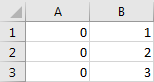Step 1: In any blank cell, enter the formula =MEDIAN(A1:B3).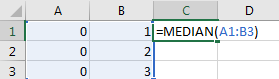Step 2: Click Enter to get the result. Verify that zero number is included in calculating.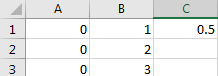## Calculate the Median for A Range Excluding Zero Number

We still use above example to calculate the median, but this time zero numbers will be ignored in calculating.

Step 1: In any blank cell, enter the formula =MEDIAN(IF(A1:B3>0,A1:B3)).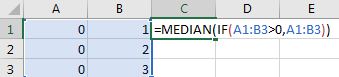Step 2: Press ‘Ctrl + Shift + Enter’ to get the result. Verify that zero number is excluded in calculating.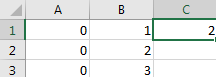## Calculate the Median for Multiple Ranges in Excel

See the table below: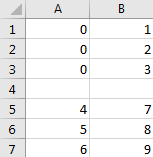You can see that there are two ranges of data in two tables. So, if we want to calculate the median for the two ranges, you can follow below steps to calculate the median.

Step 1: In any blank cell, enter the formula =MEDIAN(A1:B3,A5:B7).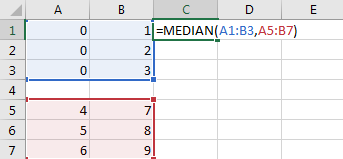If there are multiple ranges exist, we can use a comma to separate different ranges.

Step 2: Click Enter to get the result.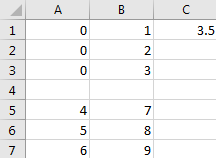### Related Functions

• Excel MEDIAN function
The Excel AVERAGE function returns the median of the given numbers. And the median is the number in the middle of a list of supplied numbers.The syntax of the MEDIAN function is as below:=MEDIAN (number1, [number2], …)….
• Excel IF function
The Excel IF function perform a logical test to return one value if the condition is TRUE and return another value if the condition is FALSE. The IF function is a build-in function in Microsoft Excel and it is categorized as a Logical Function.The syntax of the IF function is as below:= IF (condition, [true_value], [false_value])….